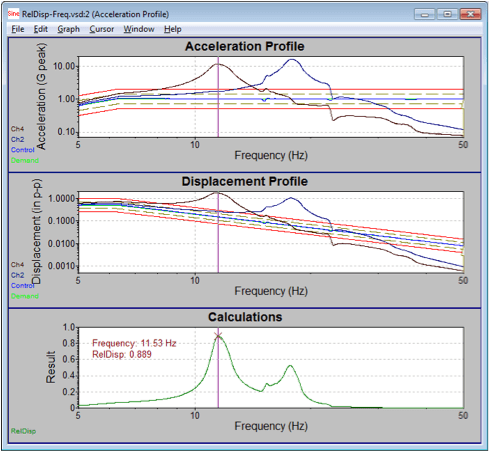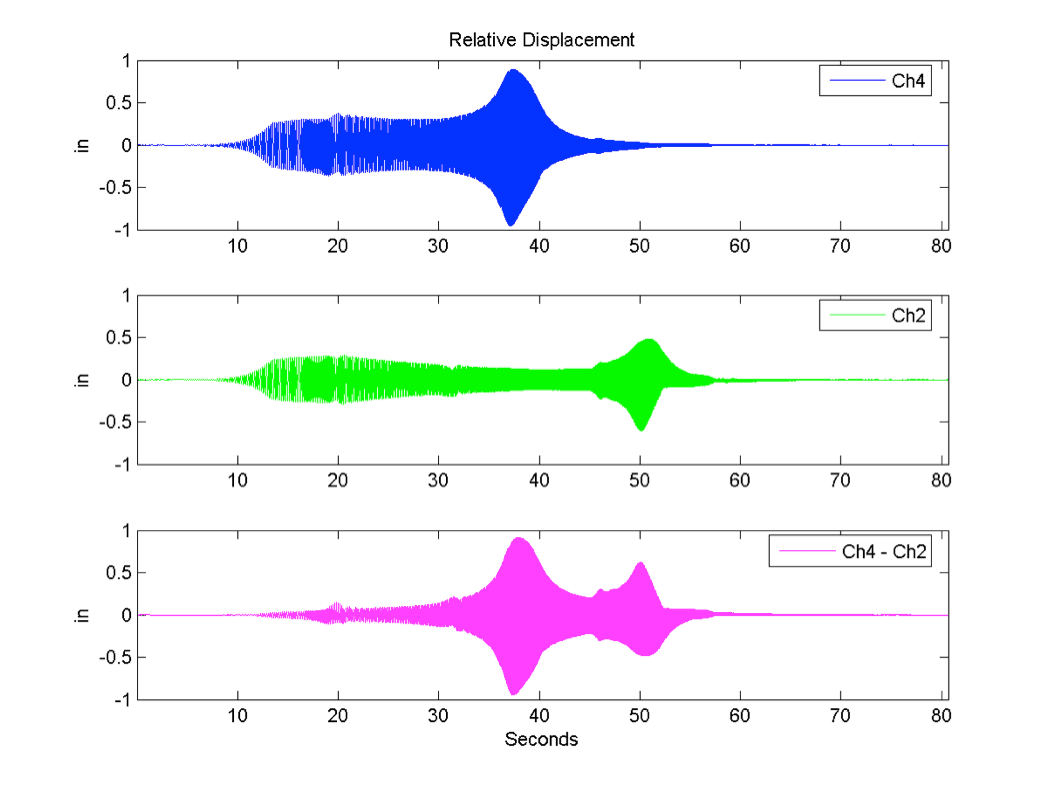# VN0009 - Computing Relative Displacement

## AbstractTwo methods of calculating the relative displacement between two channels are described.

### Question

We often get asked how to compute the relative displacement between two channels. There are two ways this can be accomplished that trade off accuracy and ease of use.

This is not just a simple matter of subtracting channel two from channel one. That only works for a pure sine tone if the channels are perfectly in phase. As an example, let’s say that channel 1 and channel 2 both are experiencing a sine tone with an amplitude of 1 inch. If the two channels are perfectly in phase, the relative displacement between the two will be zero. If they are 180 degrees out of phase, the relative displacement will be 2 inches.

CALCULATOR METHOD

In a sine test, we can filter out the harmonics and calculate the relative displacement assuming a pure sine tone. We will also need to take phase into account. We start by plotting the signal for channel 1 and channel 2 as vectors with the phase between the two channels shown as θ.The relative displacement between Ch1 and Ch2, labeled Δ, can be calculated with the following equation:

∆ = 𝐶ℎ12+ 𝐶ℎ22−2∙𝐶ℎ1∙𝐶ℎ2∙cos𝜃

To use this in VibrationVIEW you will need the Calculator feature (part # VR9606). Go to the Calculator tab on the test settings dialog and click the Insert button to add a new line. Enter the Label as “RelDisp” and enter the following equation. The source data should be Waveform.

sqrt(([PARAM:ChDisplacement1]/2)^2 + ([PARAM:ChDisplacement2]/2)^2 – 2 * ([PARAM:chDisplacement1]/2) * ([PARAM:ChDisplacement2]/2) * cos(([PARAM:ChPhase1] – [PARAM:ChPhase2])*pi/180) )Note that the [PARAM:ChDisplacement1] is divided by 2. That is because the PARAM value is peak to peak. The equation requires the amplitude. When the test is running, this calculator trace will continually calculate the relative displacement between Ch1 and Ch2. The results can either be graphed vs time or vs frequency. Click the Edit Graphand select the Calculator vs Freq. radio button. Then select the RelDisp math trace.An example of the results is shown below. In this case, the maximum relative displacement between channels 2 and 4 is 0.889 inches and occurs at 11.53 Hz.The limitation of this method is that it will only work for a sine test and the relative displacement is only calculated at the excitation frequency, other harmonics are not taken into account.

POST PROCESSING METHOD

To calculate the relative displacement across a broad frequency range, the entire waveform must be recorded and then a third party tool such as MatLab or Octave must be used to calculate the relative displacement.

If the data is recorded using a displacement transducer then a simple point by point subtraction can be used to calculate the relative displacement. Usually however, the recorded data will come from accelerometers. In that case the data will need to be converted from acceleration to displacement. A way to convert from acceleration to displacement is to double-integrate using cumulative sum. This method is prone to random walk so all you see is the very low frequency random noise. A better way to do the conversion is to apply a 2nd order low-pass-filter with a corner frequency below the minimum frequency of interest. To do this would require the Signal Processing Toolkit for MatLab.

The above script was run on a recording taken while the previous example was running and the results are shown below. There are some differences in the output. The calculator method only shows the relative displacement. The post processing method also shows the two channels in relationship to each other. The other difference is that the post processing method takes all the frequencies into account when calculating the relative displacement.The graph above shows the displacement vs time of channel 4, channel 2 and the relative displacement between the two. It may not be clear from the graph above, but the maximum and minimum values do not occur at the same time. The maximum value of 0.91 inches occurs at 37.9 seconds and the minimum value of -0.94 inches occurs at 37.5 seconds.

maxRelDisp = 0.9134 maxTime = 37.9397 maxFreq = 11.4167
minRelDisp = -0.9440 minTime = 37.4541 minFreq = 11.2219

For this test, the COLA was configured to output DC voltage proportional to the sine test frequency and looped back to channel 3. Channel 3 was configured as 1000 mv/Volts with a DC signal. That allows us to see that the frequency at the time of the maximum relative displacement was 11.4 Hz. The minimum relative displacement was at 11.2 Hz.

In this case, the difference is rather insignificant and is only about 5.5 cycles but it shows that the maximum and minimum relative displacement does not have to occur at the same time.

This example uses a sine test, but this method does work with a random test as well.